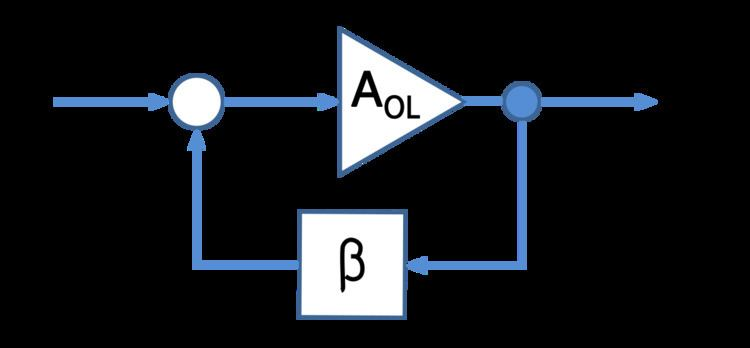# Loop gain

Updated onIn electronics and control system theory, loop gain is the sum of the gain, expressed as a ratio or in decibels, around a feedback loop. Feedback loops are widely used in electronics in amplifiers and oscillators, and more generally in both electronic and nonelectronic industrial control systems to control industrial plant and equipment. The concept is also used in biology. In a feedback loop, the output of a device, process or plant is sampled and applied to alter the input, to better control the output. The loop gain, along with the related concept of loop phase shift, determines the behavior of the device, and particularly whether the output is stable, or unstable, which can result in oscillation. The importance of loop gain as a parameter for characterizing electronic feedback amplifiers was first recognized by Heinrich Barkhausen in 1921, and was developed further by Hendrik Wade Bode and Harry Nyquist at Bell Labs in the 1930s.

A block diagram of an electronic amplifier with negative feedback is shown at right. The input signal is applied to the amplifier with open-loop gain A and amplified. The output of the amplifier is applied to a feedback network with gain β, and subtracted from the input to the amplifier. The loop gain is calculated by imagining the feedback loop is broken at some point, and calculating the net gain if a signal is applied. In the diagram shown, the loop gain is the product of the gains of the amplifier and the feedback network, −Aβ. The minus sign is because the feedback signal is subtracted from the input.

The gains A and β, and therefore the loop gain, generally vary with the frequency of the input signal, and so are usually expressed as functions of the angular frequency ω in radians per second. It is often displayed as a graph with the horizontal axis frequency ω and the vertical axis gain. In amplifiers, the loop gain is the difference between the open-loop gain curve and the closed-loop gain curve (actually, the 1/β curve) on a dB scale.

## References

Similar Topics
Chammak Challo (film)
Gilles Wife